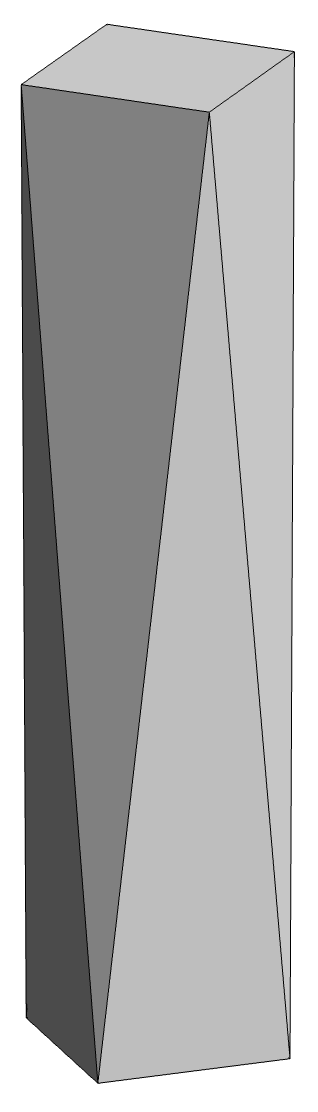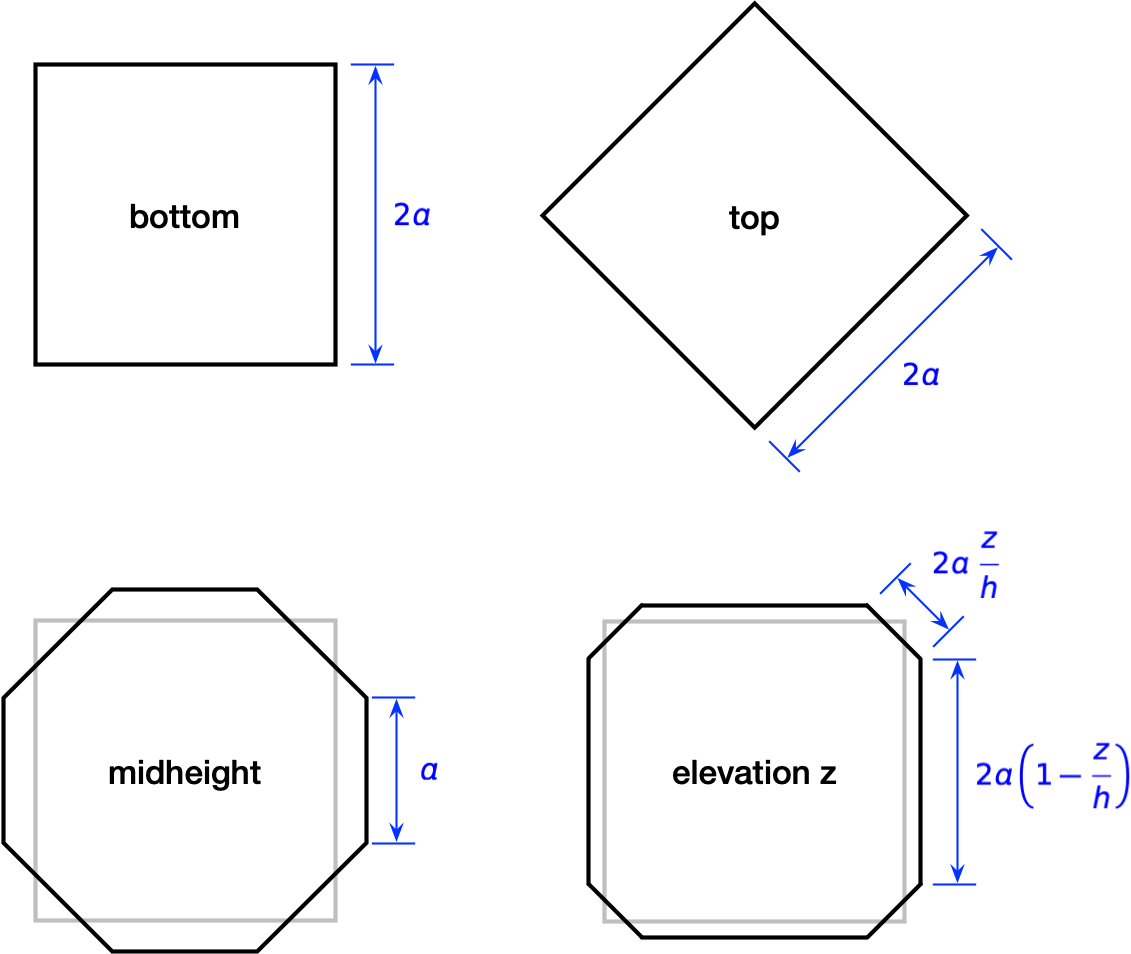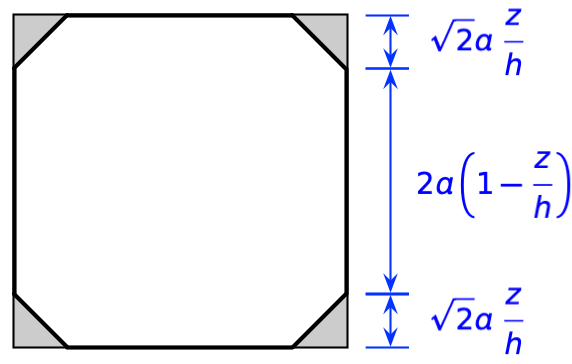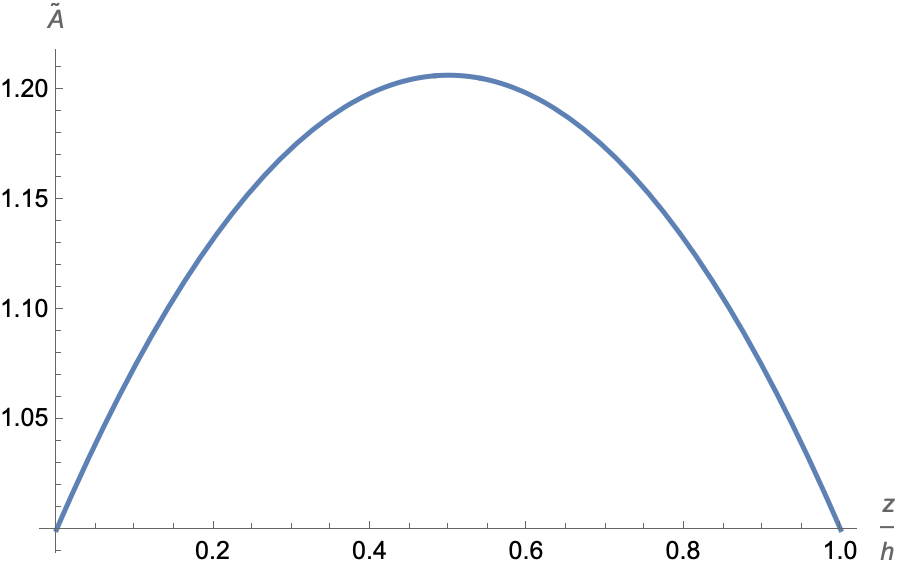# Antiprism

A couple of days ago, Matt Parker posted this video about antiprisms.

It ends with Matt and Laura Taalman calculating the volume enclosed by the upper portion of the One World Trade Center tower and getting a very neat and compact solution:

$V=56AbasehV = \frac{5}{6} A_{base} h$

This works out so nicely because the tower’s antiprism is tapered such that its roof has half the area of its base. Watch the video for the details.

What I want to talk about here is the question that comes up earlier in the video: Is the volume of a square-ended antiprism less than, greater than, or equal to the volume of a square-ended prism of the same base and height?

Since I’m sure you watched the video, I don’t have to show you what a square-ended antiprism looks like, but here it is anyway:The orientation of the square at the top is turned 45° to that of the square at the bottom, and the top and bottom are connected by triangular faces.

Matt’s reasoning regarding the volume of this shape relative to a prism with the same top, bottom, and height is both impeccable and familiar. He considers the cross-section of the antiprism at midheight, and recognizes that it’s an octagon with the same perimeter as the squares at the bottom and top. Since such an octagon has an area greater than the squares, the volume of the antiprism must be greater than the volume of the prism.

What made this familiar to me was that I did this same octagon/square comparison last year. The ratio of the octagon area to the square area is

$12(1+2)≈65\frac{1}{2} \left( 1 + \sqrt{2} \right) \approx \frac{6}{5}$

But that’s where Matt and Laura stop with the antiprism and move on to the tapered antiprism of One World Trade Center. I wanted them to follow through with the ratio of the volumes of the antiprism and prism. Since they didn’t, I will.

I didn’t try anything clever. I started by looking at the cross-sections at various heights.The key was the diagram in the lower right corner, which gives the dimensions of the non-regular octagon at an arbitrary height z above the base. You can see how it matches the dimensions of the other three cross-sections for z values of 0, h, and h/2.

To get the area of the arbitrary octogon, I redrew the figure this way and subtracted the areas of the four gray triangles from that of the large square.$A=[2a(1−zh)+22azh]2−4[12(2azh)2]A = \left[ 2 a \left(1 - \frac{z}{h} \right) + 2 \sqrt{2} a \frac{z}{h} \right]^2 - 4 \left[ \frac{1}{2} \left( \sqrt{2} a \frac{z}{h} \right)^2 \right]$

Simplifying, we get

$A=4a2[1+2(2−1)zh−2(2−1)(zh)2]A = 4 a^2 \left[ 1 + 2 \left( \sqrt{2} - 1 \right) \frac{z}{h} - 2 \left( \sqrt{2} - 1 \right) \left( \frac{z}{h} \right)^2 \right]$

The ratio of this area to that of the squares at the top and bottom is

$A~=A4a2=1+2(2−1)zh−2(2−1)(zh)2\widetilde{A} = \frac{A}{4 a^2} = 1 + 2 \left( \sqrt{2} - 1 \right) \frac{z}{h} - 2 \left( \sqrt{2} - 1 \right) \left( \frac{z}{h} \right)^2$

which plots as this parabola:Note that this matches our expectations of 1 at the top and bottom and about 1.2 at midheight.

To get the volume, we integrate the area from bottom to top:

$V=∫0hAdz=4a2∫0hA~dzV = \int_0^h A\; dz = 4 a^2 \int_0^h \widetilde{A}\; dz$ $V=4a2h(2+23)=(2+23)AbasehV = 4 a^2 h \left( \frac{2 + \sqrt{2}}{3} \right) = \left( \frac{2 + \sqrt{2}}{3} \right) A_{base} h$

which is about 14% more than the volume of the prism. This is not as simple as the formula Matt and Laura got for the tapered antiprism, but it’s still fairly compact.